# R graphics q R has several graphics packages

• Slides: 20
Download presentationR graphics q R has several graphics packages q The plotting functions are quick and easy to use q We will cover: § Bar charts – frequency, proportion § Pie charts § Histograms § Box plots § Scatter plots q Explore further on your own - R help, demo(graphics)Bar charts q A bar chart draws a bar with a height proportional to the count in the table q The height could be given by the frequency, or the proportion, where the graph will look the same, but the scales may be different q Use scan() to read in the data from a file or by typing q Try ? scan for more information q Usage is simple: type in the data. It stops adding data when you enter a blank row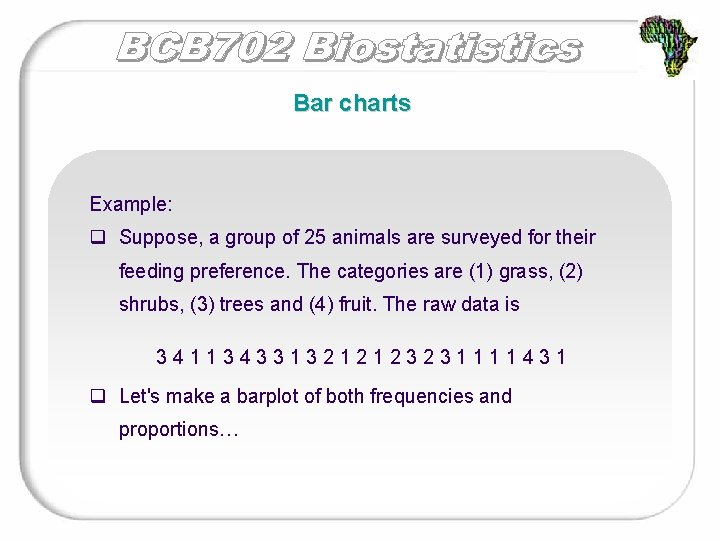Bar charts Example: q Suppose, a group of 25 animals are surveyed for their feeding preference. The categories are (1) grass, (2) shrubs, (3) trees and (4) fruit. The raw data is 3411343313212123231111431 q Let's make a barplot of both frequencies and proportions…Bar chart - frequency Example: Feeding preference > feed = scan() 1: 3 4 1 1 3 4 3 3 1 3 2 1 2 3 1 1 4 3 1 26: Read 25 items > barplot(table(feed)) Note: barplot(feed) is not correct. Use table command to create summarized data, and the result of this is sent to barplot creating the barplot of frequencies FrequencyBar chart - proportion Example cont… > barplot(table(feed)/length(feed)) > table(feed)/length(feed) feed 1 2 3 4 0. 40 0. 16 0. 32 0. 12 # divide by n for proportion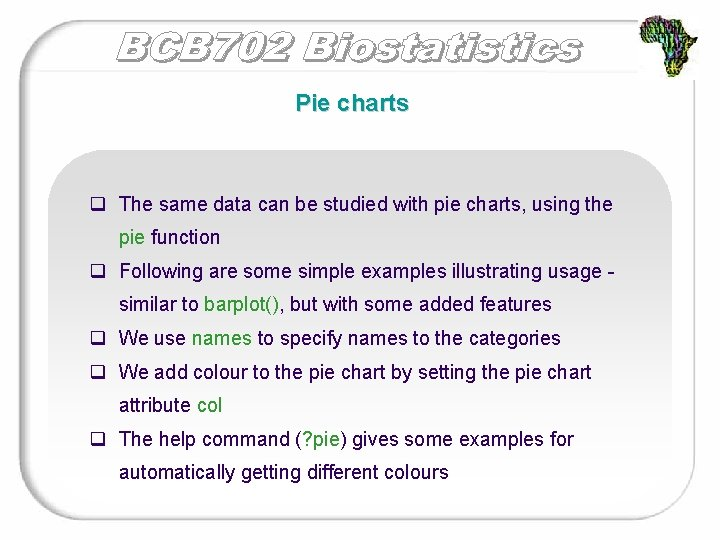Pie charts q The same data can be studied with pie charts, using the pie function q Following are some simple examples illustrating usage similar to barplot(), but with some added features q We use names to specify names to the categories q We add colour to the pie chart by setting the pie chart attribute col q The help command (? pie) gives some examples for automatically getting different colours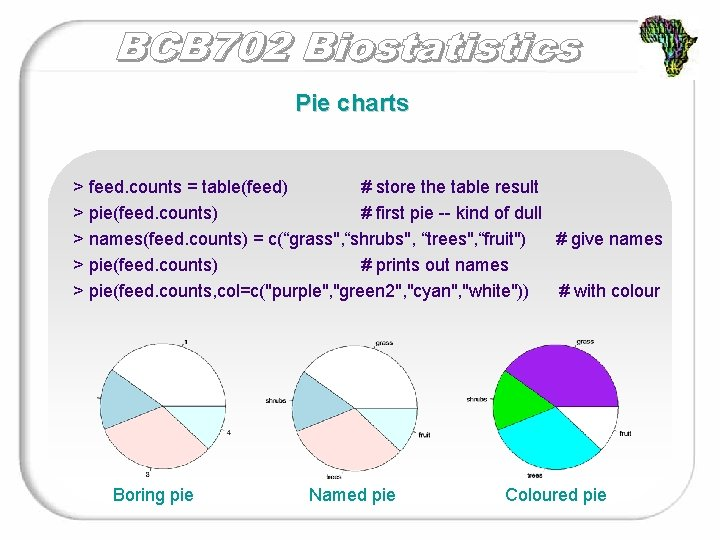Pie charts > feed. counts = table(feed) # store the table result > pie(feed. counts) # first pie -- kind of dull > names(feed. counts) = c(“grass", “shrubs", “trees", “fruit") # give names > pie(feed. counts) # prints out names > pie(feed. counts, col=c("purple", "green 2", "cyan", "white")) # with colour Boring pie Named pie Coloured pie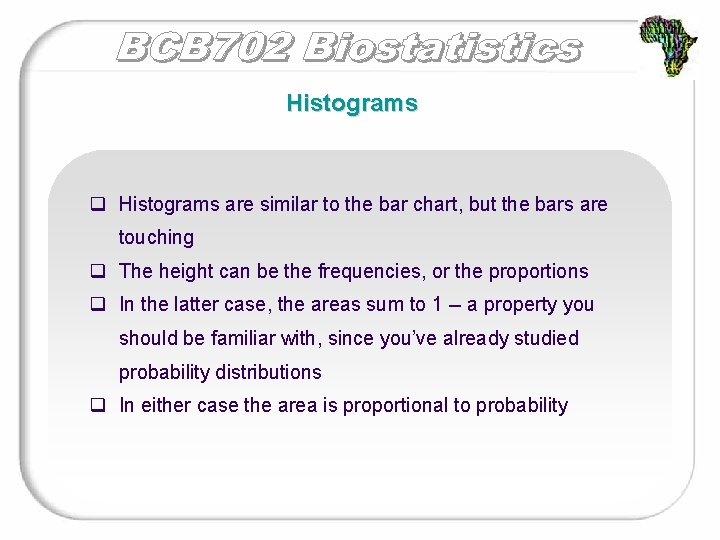Histograms q Histograms are similar to the bar chart, but the bars are touching q The height can be the frequencies, or the proportions q In the latter case, the areas sum to 1 -- a property you should be familiar with, since you’ve already studied probability distributions q In either case the area is proportional to probability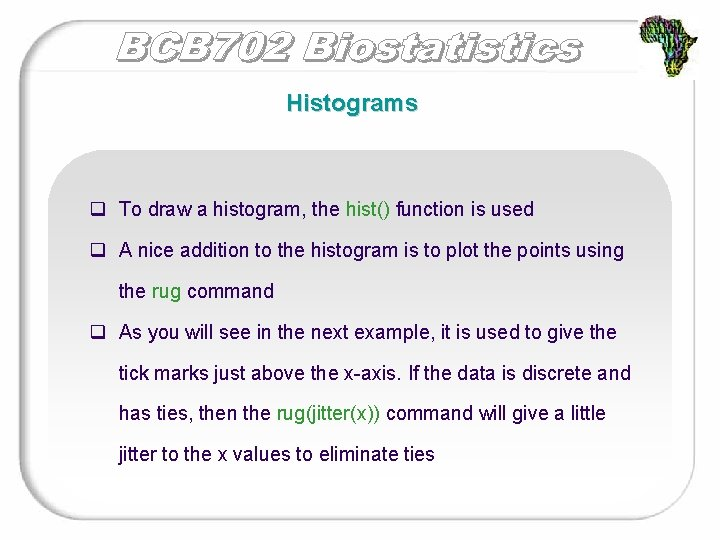Histograms q To draw a histogram, the hist() function is used q A nice addition to the histogram is to plot the points using the rug command q As you will see in the next example, it is used to give the tick marks just above the x-axis. If the data is discrete and has ties, then the rug(jitter(x)) command will give a little jitter to the x values to eliminate tiesHistograms Example: Suppose a lecturer recorded the number of hours that 15 students spent studying for their exams during one week 29. 6 28. 2 19. 6 13. 7 13. 0 7. 8 3. 4 2. 0 1. 9 1. 0 0. 7 0. 4 0. 3 Enter the data: > a=scan() 1: 29. 6 28. 2 19. 6 13. 7 13. 0 7. 8 3. 4 2. 0 1. 9 1. 0 0. 7 0. 4 0. 3 16: Read 15 itemsHistograms Draw a histogram: > hist(a) # frequencies > hist(a, probability=TRUE) # proportions (or probabilities) > rug(jitter(a)) # add tick marks NULL histogram of frequencies (default) Note different y-axis preferred histogram of proportions (total area = 1)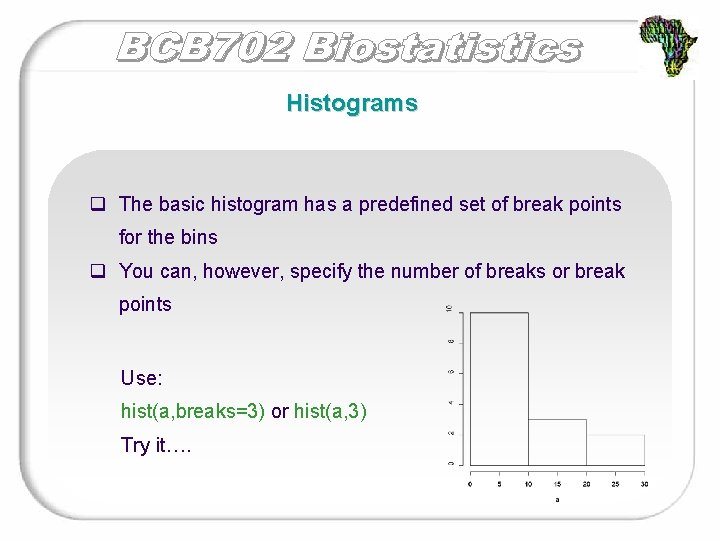Histograms q The basic histogram has a predefined set of break points for the bins q You can, however, specify the number of breaks or break points Use: hist(a, breaks=3) or hist(a, 3) Try it….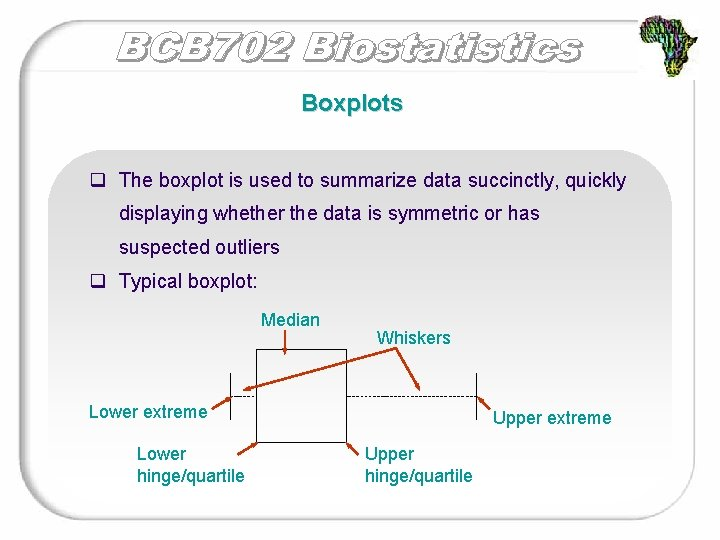Boxplots q The boxplot is used to summarize data succinctly, quickly displaying whether the data is symmetric or has suspected outliers q Typical boxplot: Median Whiskers Lower extreme Lower hinge/quartile Upper extreme Upper hinge/quartile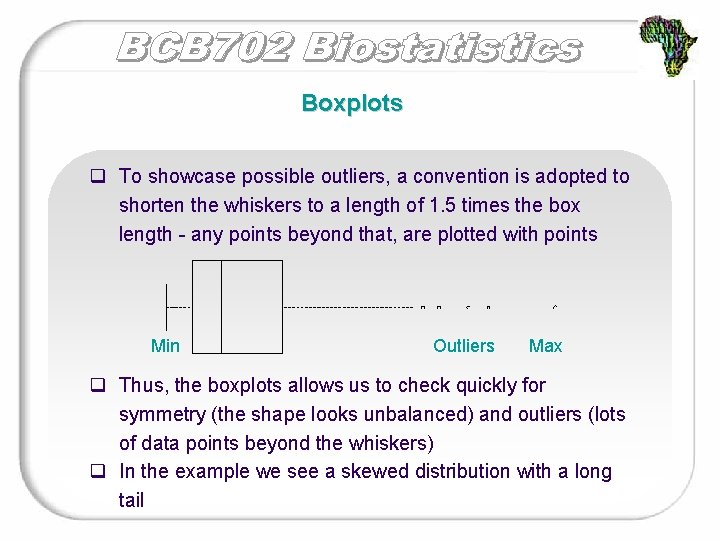Boxplots q To showcase possible outliers, a convention is adopted to shorten the whiskers to a length of 1. 5 times the box length - any points beyond that, are plotted with points Min Outliers Max q Thus, the boxplots allows us to check quickly for symmetry (the shape looks unbalanced) and outliers (lots of data points beyond the whiskers) q In the example we see a skewed distribution with a long tail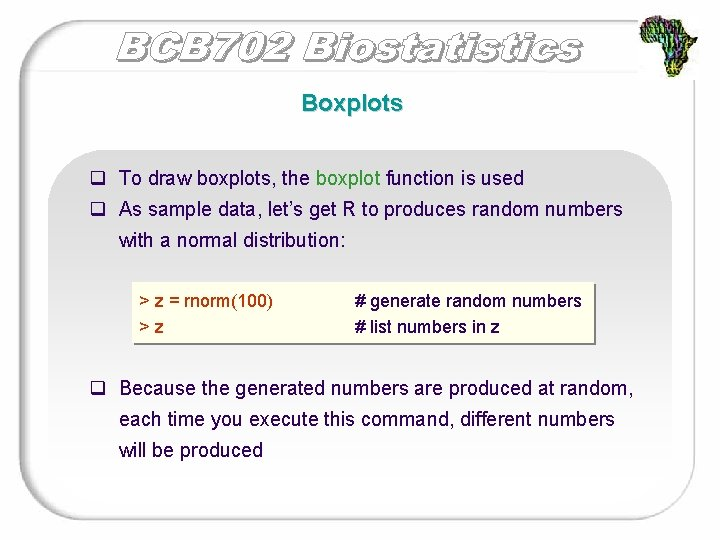Boxplots q To draw boxplots, the boxplot function is used q As sample data, let’s get R to produces random numbers with a normal distribution: > z = rnorm(100) >z # generate random numbers # list numbers in z q Because the generated numbers are produced at random, each time you execute this command, different numbers will be produced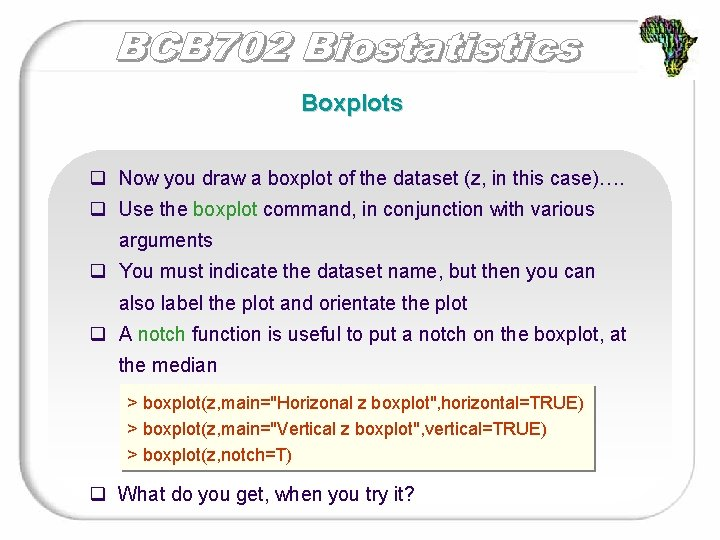Boxplots q Now you draw a boxplot of the dataset (z, in this case)…. q Use the boxplot command, in conjunction with various arguments q You must indicate the dataset name, but then you can also label the plot and orientate the plot q A notch function is useful to put a notch on the boxplot, at the median > boxplot(z, main="Horizonal z boxplot", horizontal=TRUE) > boxplot(z, main="Vertical z boxplot", vertical=TRUE) > boxplot(z, notch=T) q What do you get, when you try it?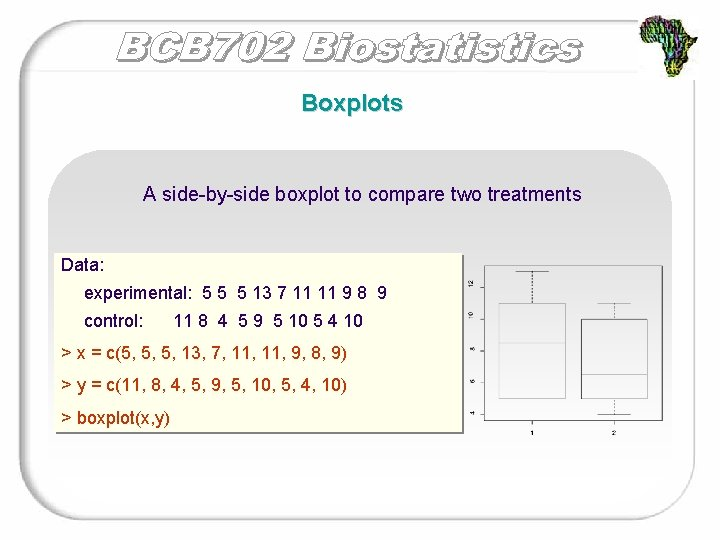Boxplots A side-by-side boxplot to compare two treatments Data: experimental: 5 5 5 13 7 11 11 9 8 9 control: 11 8 4 5 9 5 10 5 4 10 > x = c(5, 5, 5, 13, 7, 11, 9, 8, 9) > y = c(11, 8, 4, 5, 9, 5, 10, 5, 4, 10) > boxplot(x, y)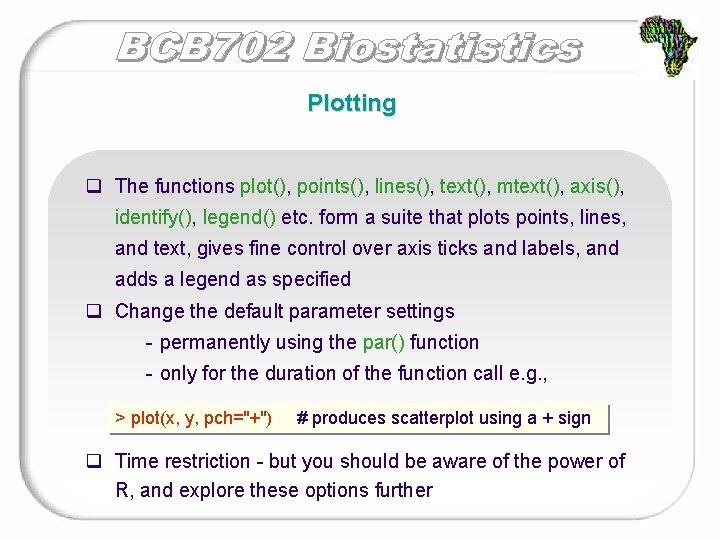Plotting q The functions plot(), points(), lines(), text(), mtext(), axis(), identify(), legend() etc. form a suite that plots points, lines, and text, gives fine control over axis ticks and labels, and adds a legend as specified q Change the default parameter settings - permanently using the par() function - only for the duration of the function call e. g. , > plot(x, y, pch="+") # produces scatterplot using a + sign q Time restriction - but you should be aware of the power of R, and explore these options furtherScatter plots q The plot function will draw a scatter plot q Additional descriptions of the plot can be included q Using the data from the previous example, draw some scatter plots…. > plot(x) > plot(x, y) > plot(y, x) # change axis > plot(x, pch=c(2, 4)) # print character > plot(x, col=c(2, 4)) # adds colour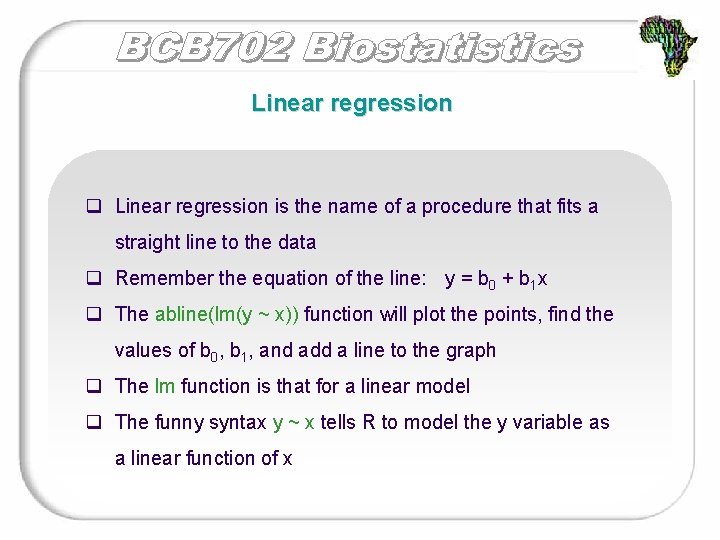Linear regression q Linear regression is the name of a procedure that fits a straight line to the data q Remember the equation of the line: y = b 0 + b 1 x q The abline(lm(y ~ x)) function will plot the points, find the values of b 0, b 1, and add a line to the graph q The lm function is that for a linear model q The funny syntax y ~ x tells R to model the y variable as a linear function of x